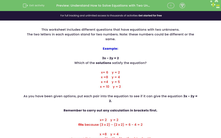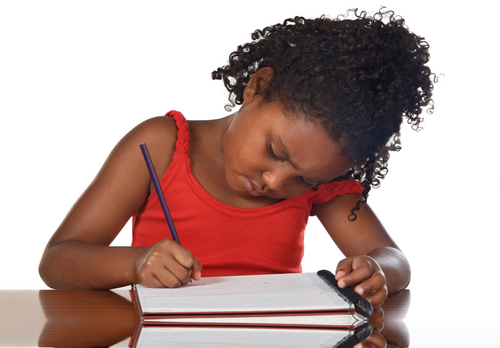# Understand How to Solve Equations with Two Unknowns

In this worksheet, students will be asked to solve a range of harder equations with two unknowns.Key stage:  KS 2

Curriculum topic:   Algebra

Curriculum subtopic:   Use Equations

Popular topics:   Algebra worksheets

Difficulty level:#### Worksheet Overview

This activity includes different questions that have equations with two unknowns.

The two letters in each equation stand for two numbers. Note: these numbers could be different or the same.

Example:

3x - 2y = 2

Which of the solutions satisfy the equation?

x =  6    y = 2

x = 8    y = 4

x = 4    y = 5

x = 10    y = 2

As you have been given options, put each pair into the equation to see if it can give the equation 3x - 2y = 2.

Remember to carry out any calculation in brackets first.

x = 2    y = 2

fits because (3 x 2) - (2 x 2) = 6 - 4 = 2

x = 8    y = 4

does not fit because

(3 x 8) - (2 x 4) = 24 - 8 = 16

x = 4    y = 5

fits because (3 x 4) - (2 x 5) = 12 - 10 = 2

x = 10    y = 2

does not fit because

(3 x 10) - (2 x 2) = 30 - 4 = 26

Remember to use a sensible process of trial and error when solving equations with two unknowns.

Let's start the questions now.### What is EdPlace?

We're your National Curriculum aligned online education content provider helping each child succeed in English, maths and science from year 1 to GCSE. With an EdPlace account you’ll be able to track and measure progress, helping each child achieve their best. We build confidence and attainment by personalising each child’s learning at a level that suits them.

Get started